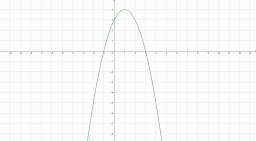# Intersections 62784

A quadratic function is given: y = -x² + 2x + 3
a) determine the intersections with the x, y axis
and peak V

b) draw a graph and describe

c) for which x applies
f (x) = 3

Result

Y0 = (Correct answer is: [2,0])Y1 = (Correct answer is: [1,0])X0 = (Correct answer is: [0,3])V = (Correct answer is: [1,4])x1 =  2
x2 =  0

### Step-by-step explanation:Did you find an error or inaccuracy? Feel free to write us. Thank you!

Tips for related online calculators
Line slope calculator is helpful for basic calculations in analytic geometry. The coordinates of two points in the plane calculate slope, normal and parametric line equation(s), slope, directional angle, direction vector, the length of the segment, intersections of the coordinate axes, etc.
Are you looking for help with calculating roots of a quadratic equation?
Do you have a linear equation or system of equations and looking for its solution? Or do you have a quadratic equation?

#### Grade of the word problem:

We encourage you to watch this tutorial video on this math problem: